7 篇文章 0 订阅

# 导包

import numpy as np
import pandas as pd
import matplotlib.pyplot as plt
import seaborn as sns

# 正常显示中文标签
plt.rcParams['font.sans-serif'] = ['SimHei']
# 自动适应布局
plt.rcParams.update({'figure.autolayout': True})
# 正常显示负号
plt.rcParams['axes.unicode_minus'] = False


# matplotlib基本参数

axex: 设置坐标轴边界和表面的颜色、坐标刻度值大小和网格的显示

figure: 控制dpi、边界颜色、图形大小、和子区( subplot)设置

font: 字体集（font family）、字体大小和样式设置

grid: 设置网格颜色和线性

legend: 设置图例和其中的文本的显示

line: 设置线条（颜色、线型、宽度等）和标记

patch: 是填充2D空间的图形对象，如多边形和圆。控制线宽、颜色和抗锯齿设置等。

savefig: 可以对保存的图形进行单独设置。例如，设置渲染的文件的背景为白色。

xticks和yticks: 为x,y轴的主刻度和次刻度设置颜色、大小、方向，以及标签大小。

legend loc位置 参数

best 0

upper right / 右上方 1

upper left / 左上方 2

lower left / 左下方 3

lower right / 右下方 4

right / 右 5

Center left / 左 6

Center left / 右 7

Center lower / 中下方 8

Center upper / 中上方 9

Center upper / 中上方 9

Center / 中 10

fig, ax = plt.subplots(1, 2, figsize = (6, 4), dpi=90)
plt.subplot(1, 2, 1)
plt.title('this is title')
plt.xlabel('X 轴')
plt.ylabel('Y 轴')
plt.xlim(0, 1)
plt.ylim(-1, 1)
plt.xticks([])
plt.yticks([-1, 0, 1],['差','一般','好'], rotation=45)
data1 = np.linspace(-1, 10, 100)
data2 = np.arange(0, 1, 0.1)
plt.plot(data1, data1**2, 'g*--')
plt.plot(data2, data2*(-1), 'yo-.')
plt.legend(['y1', 'y2'], loc='center', frameon=False, edgecolor='red')
# edgecolor设置图例背景，若无边框，则无效

plt.subplot(1, 2, 2)
plt.title('title sin')
plt.xlabel('I am X 轴')
plt.ylabel('I am Y 轴')
plt.xlim(0, 1)
plt.ylim(0, 1)
plt.xticks([0, 0.5, 1],['差','一般','好'], rotation=-45)
plt.yticks([])
data = np.linspace(-1, 10, 100)
plt.plot(data, np.sin(data), 'bd--', label='sin')
plt.legend(loc='best', edgecolor='y')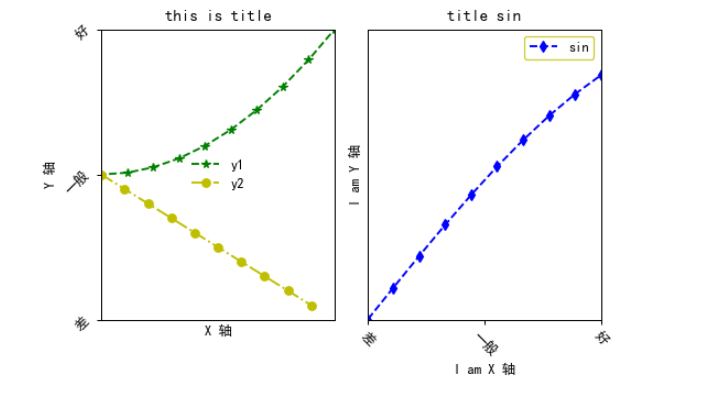fig = plt.figure()
ax1.plot(np.random.randn(30).cumsum(), 'k+:', label='random')
ax1.set_xticks([0, 10, 20, 30])
ax1.set_xticklabels(['a', 'b', 'c', 'd'], rotation=30, fontsize='large')
ax1.legend()

ax2 = plt.gca()
ax2.spines['right'].set_color('none')
ax2.spines['top'].set_color('none')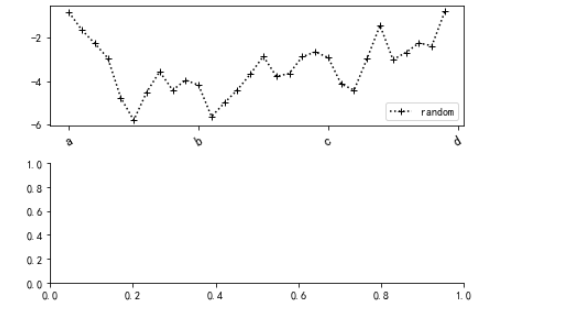## 折线图

plt.plot(x, y, color=‘颜色’, linstyle, marker, alpha, label=‘标签’)

color = {‘b’:‘蓝色’, ‘g’:‘绿色’, ‘r’:‘红色’, ‘c’:‘青色’, ‘m’:‘品红’, ‘y’:‘黄色’, ‘k’:‘黑色’, ‘w’:‘白色’}

linstyle = {’-’:‘实线’, ‘–’:‘长虚线’, ‘-.’:‘点线’, ‘:’:‘短虚线’}

‘o’ 圆圈

‘.’ 点

‘D’ 菱形

‘s’ 正方形

‘h’ 六边形1

‘*’ 星号

‘H’ 六边形2

‘d’ 小菱形

‘_’ 水平线

‘v’ 一角朝下的三角形

‘8’ 八边形

‘<’ 一角朝左的三角形

‘p’ 五边形

‘>’ 一角朝右的三角形

‘,’ 像素

‘^’ 一角朝上的三角形

‘+’ 加号

‘\ ‘ 竖线

‘None’,’’,’ ‘ 无

‘x’ X

## 绘制直方图 hist(), plt.bar()

plt.bar(left, height, width, color, align, yerr))

left为x轴的位置序列，一般采用arange函数产生一个序列；

height为y轴的数值序列，也就是柱形图的高度，一般就是我们需要展示的数据；

width为柱形图的宽度，一般这是为1即可；
color为柱形图填充的颜色;

align设置plt.xticks()函数中的标签的位置；

yerr让柱形图的顶端空出一部分。

color设置柱状的颜色

alpha 设置柱状填充颜色的透明度 大于0 小于等于1

linewidth 线条的宽度

job_data = [470, 443, 240, 207, 202, 184, 151, 136, 124, 118]
how_many = [2620,1841,640,790,589,538,408,425,493,550]
labels =["北京","上海","深圳","郑州","广州","成都","杭州","长沙","武汉","大连"]
xlocation =  np.linspace(1, len(job_data) * 0.6, len(job_data)) #len(data个序列)
print(xlocation)

fig = plt.figure(figsize=(6, 6))
fig.tight_layout()
#tight_layout会自动调整子图参数，使之填充整个图像区域。这是个实验特性，可能在一些情况下不工作。它仅仅检查坐标轴标签、刻度标签以及标题的部分。
rects01 = ax1.bar(xlocation, job_data, width=0.2, color='darkgoldenrod', linewidth=1, alpha=0.8)
rects02 = ax1.bar(xlocation+0.2, how_many, width=0.2, color='seagreen', linewidth=1, alpha=0.8)
plt.xticks(xlocation+0.15,labels, fontsize=12 ,rotation = 20)
ax1.legend(['职位数量', '招聘人数'], fontsize=10)
# 添加数据标签
for r1,r2 ,amount01,amount02 in zip(rects01, rects02,job_data,how_many):
h01 = r1.get_height()
h02 = r2.get_height()
plt.text(r1.get_x(), h01, amount01, fontsize=13, va ='bottom')  # 添加职位数量标签
plt.text(r2.get_x(), h02 , amount02, fontsize=13, va='bottom')  # 添加招聘人数

data = np.array([[10., 30., 19., 22.],
[5., 18., 15., 20.],
[4., 6., 3., 5.]])
color_list = ['b', 'g', 'r']
X = np.arange(data.shape)
print('X:', X)
for i in range(data.shape):
ax2.bar(X, data[i], width=0.2, bottom=np.sum(data[:i], axis=0), color=color_list[i%len(color_list)])
# plt.savefig('zhifangtu.png', dpi=120, bbox_inches='tight')

[1.         1.55555556 2.11111111 2.66666667 3.22222222 3.77777778
4.33333333 4.88888889 5.44444444 6.        ]
X: [0 1 2 3]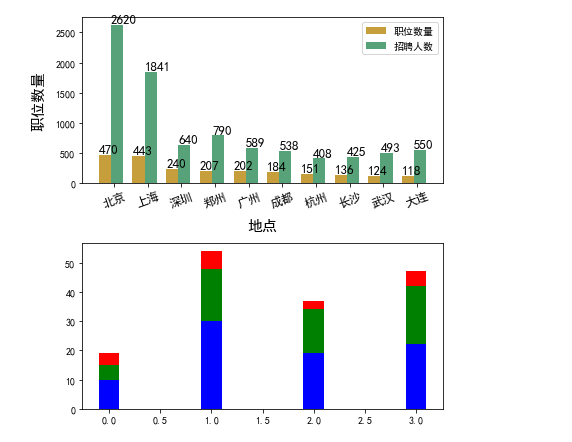## 绘制水平方向的柱状图

a.plot(kind=‘barh’)

fig = plt.figure()
fig.tight_layout()
y = [1, 2, 3, 4, 5]
width=[9, 8, 7, 6, 5]
ax1.barh(y, width, color='y')

df = pd.DataFrame(np.random.rand(5, 3), columns=['a', 'b', 'c'])
df.plot(kind='barh', color=['y', 'b', 'g'], ax=ax2)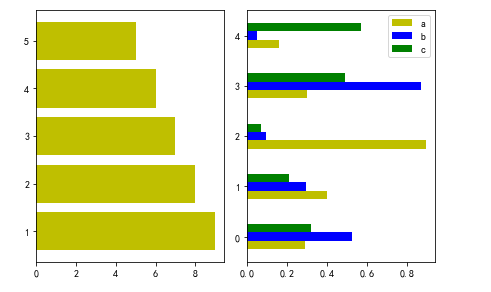## 饼图

def pie(x, explode=None, labels=None, colors=None, autopct=None,
center=(0, 0), frame=False, rotatelabels=False, hold=None, data=None)

x :(每一块)的比例，如果sum(x) > 1会使用sum(x)归一化；

labels :(每一块)饼图外侧显示的说明文字；

explode :(每一块)离开中心距离；

startangle :起始绘制角度,默认图是从x轴正方向逆时针画起,如设定=90则从y轴正方向画起；

labeldistance :label标记的绘制位置,相对于半径的比例，默认值为1.1, 如<1则绘制在饼图内侧；

autopct :控制饼图内百分比设置,可以使用format字符串或者format function
'%1.1f’指小数点前后位数(没有用空格补齐)；

pctdistance :类似于labeldistance,指定autopct的位置刻度,默认值为0.6；

wedgeprops ：字典类型，可选参数，默认值：None。参数字典传递给wedge对象用来画一个饼图。例如：wedgeprops={‘linewidth’:3}设置wedge线宽为3。

textprops ：设置标签（labels）和比例文字的格式；字典类型，可选参数，默认值为：None。传递给text对象的字典参数。

center ：浮点类型的列表，可选参数，默认值：(0,0)。图标中心位置。

frame ：布尔类型，可选参数，默认值：False。如果是true，绘制带有表的轴框架。

rotatelabels ：布尔类型，可选参数，默认为：False。如果为True，旋转每个label到指定的角度。

counterclock：指定指针方向；布尔值，可选参数，默认为：True，即逆时针。将值改为False即可改为顺时针。

labeldistance : label绘制位置,相对于半径的比例, 如<1则绘制在饼图内侧，默认值为1.1；

x = [10,20,30,40]
labels = ['A','B','C','D']
explodes = [0, 0, 0.1, 0]
colors = ['r', 'g', 'b', 'pink']
plt.pie(x, labels=labels, explode=explodes, colors=colors, shadow=True, autopct='%1.1f%%',
textprops={'fontsize':20,'color':'black'})
plt.axis('equal')  # 将饼图显示为正圆形
plt.title("饼图详解示例")
plt.text(1,-1.2,'By:youyouyou')
# loc =  'upper right' 位于右上角
# bbox_to_anchor=[0.5, 0.5] # 外边距 上边 右边
# ncol=2 分两列
plt.show()

# plt.savefig("C:\\饼图02.png",dpi=200,bbox_inches='tight')  # 保存图表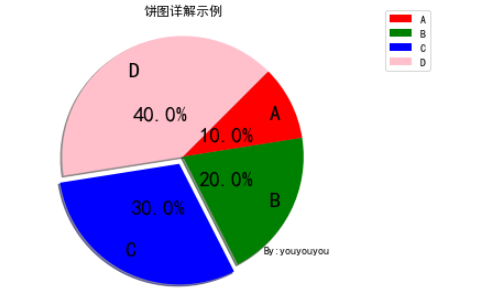## 散点图

plt.scatter(x, y, c=color)

plt.scatter(x, y, s=‘指定点的大小，可以使array’, c=‘指定点的颜色，可以使array’, marker, alpha)

x = np.arange(1, 30)
y = np.cos(x)
plt.figure(figsize=(6, 4))
plt.title('散点图')
plt.xlabel('this is x')
plt.ylabel('this is y')
for color in ['red', 'green', 'blue']:
n=500
x, y = np.random.randn(2, n)
plt.scatter(x, y, s=10, c=color, linewidths=x, label=color)
plt.legend()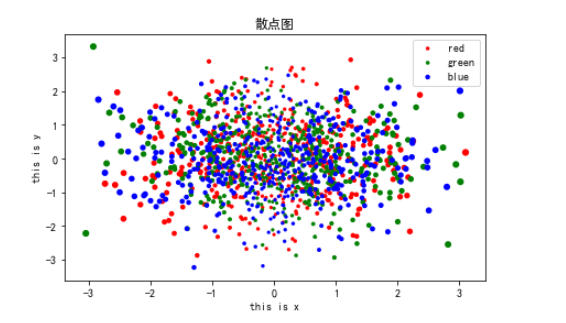## 箱线图

plt.boxplot(x,notch,sym,vert,whis,positions,widths,patch_artist,bootstrap,usermedians,conf_intervals,

meanline,showmeans,showcaps,showbox,showfliers,boxprops,labels,flierprops,medianprops,meanprops,

capprops,whiskerprops,manage_xticks,autorange,zorder,hold,data)

x 指定要绘制箱线图的数据；
showcaps 是否显示箱线图顶端和末端的两条线

notch 是否是凹口的形式展现箱线图
showbox 是否显示箱线图的箱体

sym 指定异常点的形状
showfliers 是否显示异常值

vert 是否需要将箱线图垂直摆放
boxprops 设置箱体的属性，如边框色，填充色等；

whis 指定上下须与上下四分位的距离
labels 为箱线图添加标签

positions 指定箱线图的位置
filerprops 设置异常值的属性

widths 指定箱线图的宽度
medianprops 设置中位数的属性

patch_artist 是否填充箱体的颜色；
meanprops 设置均值的属性

meanline 是否用线的形式表示均值
capprops 设置箱线图顶端和末端线条的属性

showmeans 是否显示均值
whiskerprops 设置须的属性

all_data=[np.random.normal(0,std,100) for std in range(1,4)]

#首先有图（fig），然后有轴（ax）
fig,axes = plt.subplots(nrows=1, ncols=2, figsize=(9,4))
bplot1 = axes.boxplot(all_data, vert=True, patch_artist=True)
bplot2 = axes.boxplot(all_data, notch=True, vert=True, patch_artist=True)
colors = ['pink', 'lightblue', 'lightgreen']
for bplot in (bplot1, bplot2):
for patch, color in zip(bplot['boxes'], colors):
patch.set_facecolor(color)
plt.show()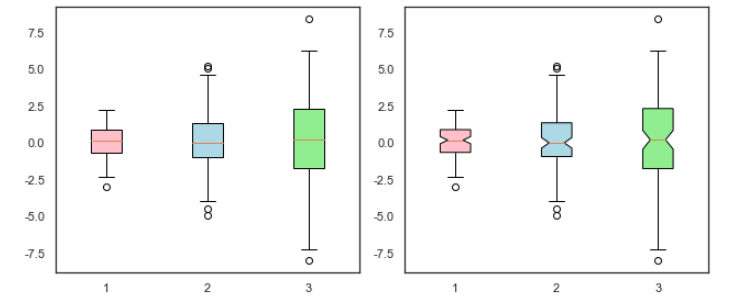# seaborn基本参数

PassengerId ：乘客Id

Survived：是否存活，存活为1，死亡为0

Pclass: 客舱等级

Name:乘客姓名

Sex:性别

Age:年龄

Sibsp:是否有兄弟姐妹

Parch: 是否有父母子女

Ticket:票编号

Fare:费用

Cabin:船舱类型

Embarked:上船地点

data = pd.read_csv('./datasets/titanic.csv')
data.info()

<class 'pandas.core.frame.DataFrame'>
RangeIndex: 891 entries, 0 to 890
Data columns (total 12 columns):
#   Column       Non-Null Count  Dtype
---  ------       --------------  -----
0   PassengerId  891 non-null    int64
1   Survived     891 non-null    int64
2   Pclass       891 non-null    int64
3   Name         891 non-null    object
4   Sex          891 non-null    object
5   Age          714 non-null    float64
6   SibSp        891 non-null    int64
7   Parch        891 non-null    int64
8   Ticket       891 non-null    object
9   Fare         891 non-null    float64
10  Cabin        204 non-null    object
11  Embarked     889 non-null    object
dtypes: float64(2), int64(5), object(5)
memory usage: 83.7+ KB


## 直方图和密度曲线图

seaborn.distplot(a, bins=None, hist=True, kde=True, rug=False, fit=None, hist_kws=None, kde_kws=None, rug_kws=None, fit_kws=None, color=None, vertical=False, norm_hist=False, axlabel=None, label=None, ax=None)

a：Series、1维数组或者列表。观察数据。如果是具有name属性的Series对象，则该名称将用于标记数据轴。

bins：matplotlib hist()的参数，或None。可选参数。直方图bins（柱）的数目，若填None，则默认使用Freedman-Diaconis规则指定柱的数目。

hist：布尔值，可选参数。是否绘制（标准化）直方图。

kde：布尔值，可选参数。是否绘制高斯核密度估计图。

rug：布尔值，可选参数。是否在横轴上绘制观测值竖线。

fit：随机变量对象，可选参数。

{hist, kde, rug, fit}_kws：字典，可选参数。底层绘图函数的关键字参数。

color：matplotlib color，可选参数。可以绘制除了拟合曲线之外所有内容的颜色。

vertical：布尔值，可选参数。如果为True，则观测值在y轴显示。

norm_hist：布尔值，可选参数。如果为True，则直方图的高度显示密度而不是计数。如果绘制KDE图或拟合密度，则默认为True。

axlabel：字符串，False或者None，可选参数。横轴的名称。如果为None，将尝试从a.name获取它；如果为False，则不设置标签。

label：字符串，可选参数。图形相关组成部分的图例标签。

ax：matplotlib axis，可选参数。若提供该参数，则在参数设定的轴上绘图。

plt.figure(figsize=(10, 4))
sns.distplot(data['Fare'], color='r', hist=True, axlabel='X轴', label='label')
plt.legend()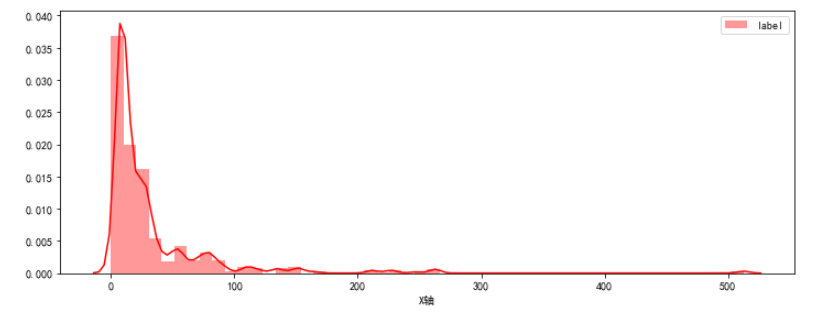plt.figure(figsize=(10, 4))
ax = sns.distplot(data['Fare'], rug=True, rug_kws={"color": "g"},
kde_kws={"color": "k", "lw": 3, "label": "KDE"},
hist_kws={"histtype": "step", "linewidth": 3, "alpha": 1, "color": "g"})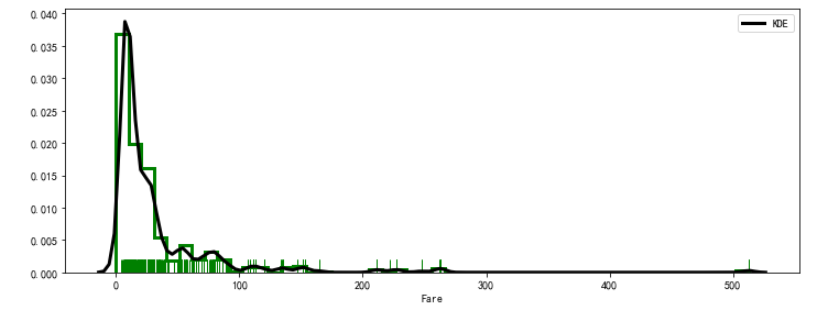### 密度曲线图

seaborn.kdeplot(data, data2=None, shade=False, vertical=False, kernel=‘gau’, bw=‘scott’, gridsize=100, cut=3, clip=None, legend=True, cumulative=False, shade_lowest=True, cbar=False, cbar_ax=None, cbar_kws=None, ax=None, **kwargs)

data：一维阵列,输入数据

**data2：一维阵列，可选。第二输入数据。如果存在，将估计双变量KDE。

vertical：布尔值，可选参数。如果为True，密度图将显示在x轴。

kernel：{‘gau’ | ‘cos’ | ‘biw’ | ‘epa’ | ‘tri’ | ‘triw’ }，可选参数要拟合的核的形状代码，双变量KDE只能使用高斯核。

bw：{‘scott’ | ‘silverman’ | scalar | pair of scalars }，可选参数用于确定双变量图的每个维的核大小、标量因子或标量的参考方法的名称。需要注意的是底层的计算库对此参数有不同的交互：statsmodels直接使用它，而scipy将其视为数据标准差的缩放因子。

gridsize：整型数据，可选参数。评估网格中的离散点数。

cut：标量，可选参数。绘制估计值以从极端数据点切割* bw。

clip：一对标量，可选参数。用于拟合KDE图的数据点的上下限值。可以为双变量图提供一对（上，下）边界。

legend：布尔值，可选参数。如果为True，为绘制的图像添加图例或者标记坐标轴。

cumulative：布尔值，可选参数。如果为True，则绘制kde估计图的累积分布。

cbar：布尔值，可选参数。如果为True并绘制双变量KDE图，为绘制的图像添加颜色条。

cbar_ax：matplotlib axes，可选参数。用于绘制颜色条的坐标轴，若为空，就在主轴绘制颜色条。

cbar_kws：字典，可选参数。fig.colorbar（）的关键字参数。

ax：matplotlib axes，可选参数。要绘图的坐标轴，若为空，则使用当前轴。

kwargs：键值对其他传递给plt.plot（）或plt.contour {f}的关键字参数，具体取决于是绘制单变量还是双变量图。

sns.set(style='white', font_scale=1.5)
sns.kdeplot(data['Fare'], color='g', shade=True, label='label', legend=True, bw=.15, cut=1)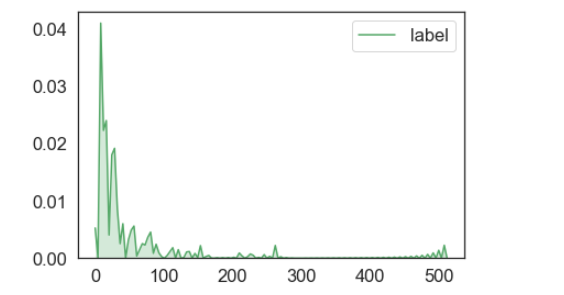sns.set()
mean, cov = [0, 2], [(1, .5), (.5, 1)]
x, y = np.random.multivariate_normal(mean, cov, size=50).T

sns.kdeplot(x, y, n_levels=30, cmap='Purples_d', cbar=True)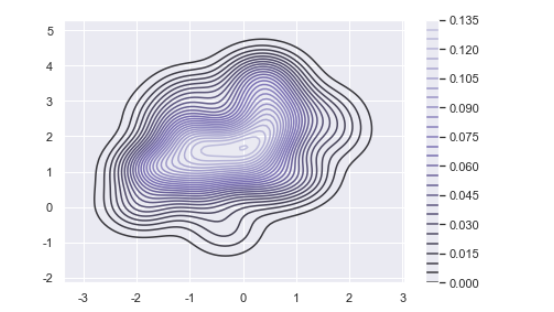iris = pd.read_csv('./datasets/iris_data.csv')
setosa = iris.loc[iris['class'] == 'Iris-setosa']
virginica = iris.loc[iris['class'] == 'Iris-versicolor']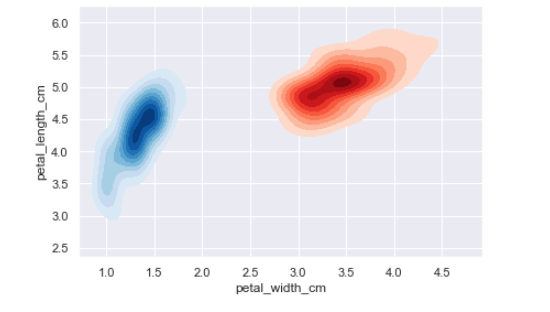### 毛毯图

seaborn.rugplot(a, height=0.05, axis=‘x’, ax=None, **kwargs)

a：vector 1D array of observations.

height：scalar, optional Height of ticks as proportion of the axis.

axis：{‘x’ | ‘y’}, optional Axis to draw rugplot on.

ax：matplotlib axes, optional Axes to draw plot into; otherwise grabs current axes.

kwargs：key, value pairings Other keyword arguments are passed to LineCollection.

sns.set_style('white')
sns.rugplot(data['Fare'], height=0.5, color='pink', label='label')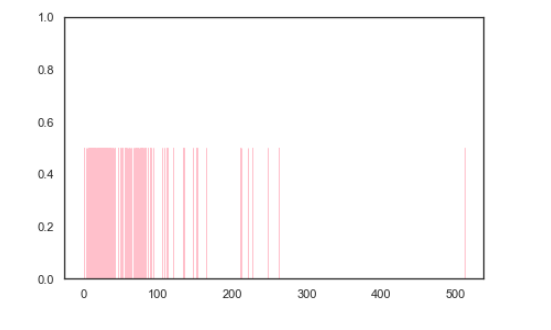## 散点图

seaborn.stripplot(x=None, y=None, hue=None, data=None, order=None, hue_order=None, jitter=True, dodge=False, orient=None, color=None, palette=None, size=5, edgecolor=‘gray’, linewidth=0, ax=None, **kwargs)

x, y, hue： 数据或矢量数据中的变量名称，可选用于绘制长格式数据的输入。查看解释示例。

data：DataFrame, 数组, 数组列表, 可选用于绘图的数据集。如果 x 和 y 不存在，则将其解释为宽格式。否则预计它将是长格式的。

order, hue_order：字符串列表，可选命令绘制分类级别，否则从数据对象推断级别。

jitter：float, True/1 是特殊的，可选要应用的抖动量（仅沿分类轴）。 当您有许多点并且它们重叠时，这可能很有用，因此更容易看到分布。您可以指定抖动量（均匀随机变量支持的宽度的一半），或者仅使用True作为良好的默认值

dodge：bool, 可选使用 hue 嵌套时，将其设置为 True 将沿着分类轴分离不同色调级别的条带。否则，每个级别的点将相互叠加。

orient：“v” | “h”, 可选图的方向（垂直或水平）。这通常是从输入变量的dtype推断出来的，但可用于指定“分类”变量何时是数字或何时绘制宽格式数据。

color：matplotlib颜色，可选所有元素的颜色，或渐变调色板的种子。

palette：调色板名称，列表或字典，可选用于色调变量的不同级别的颜色。应该是 color_palette(), 可以解释的东西，或者是将色调级别映射到matplotlib颜色的字典。

size：float, 可选标记的直径，以磅为单位。（虽然 plt.scatter 用于绘制点，但这里的 size 参数采用“普通”标记大小而不是大小^ 2，如 plt.scatter 。

edgecolor：matplotlib颜色，“灰色”是特殊的，可选的每个点周围线条的颜色。如果传递"灰色"，则亮度由用于点体的调色板决定。

linewidth：float, 可选构图元素的灰线宽度。

ax：matplotlib轴，可选返回Axes对象，并在其上绘制绘图。

ax = sns.stripplot(data['Age'])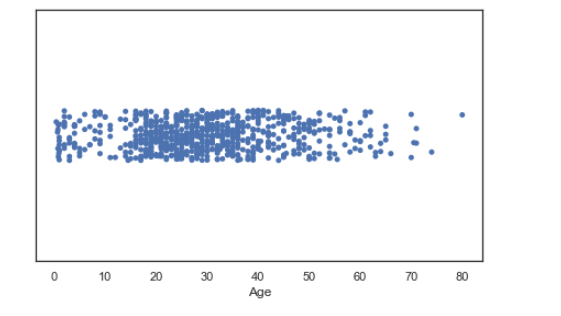ax = sns.stripplot(x='Sex', y='Age', hue='Survived', data=data, jitter=1, linewidth=1,
palette='Set2', dodge=True)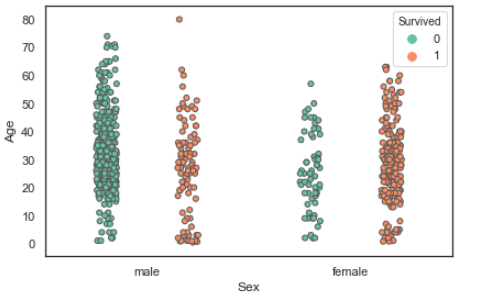ax =  sns.stripplot("Sex", "Age", hue="Survived", data=data,
size=20, marker="D", dodge=True,
edgecolor="gray", alpha=.25)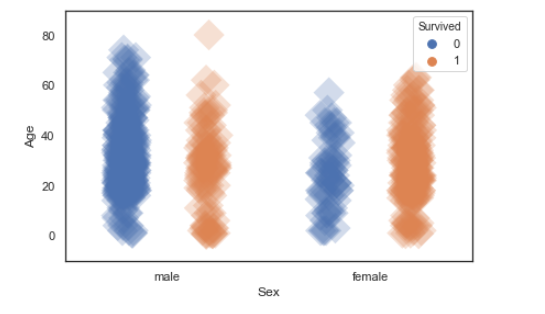sns.boxplot(x="Sex", y="Age", data=data, whis=np.inf)
sns.stripplot(x="Sex", y="Age", data=data, jitter=True, color="y")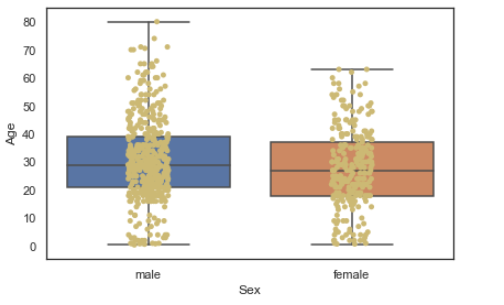sns.violinplot(x="Sex", y="Survived", data=data, inner=None, color=".8")
sns.stripplot(x="Sex", y="Survived", data=data, jitter=True)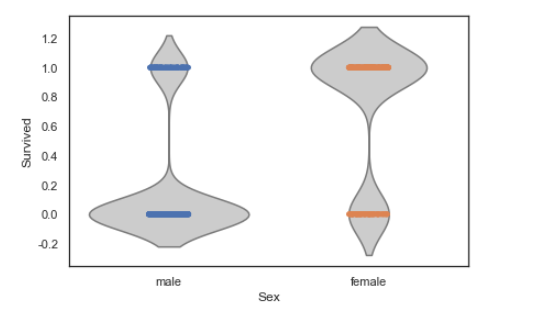### catplot()

sns.catplot(x="Sex", y="Age", col="Survived", data=data, kind="strip", jitter=True, height=4, aspect=.7)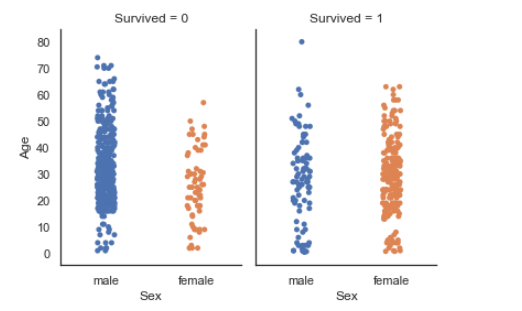### 如果需要看清每个数据点，可以使用swarmplot

seaborn.swarmplot(x=None, y=None, hue=None, data=None, order=None, hue_order=None, dodge=False, orient=None, color=None, palette=None, size=5, edgecolor=‘gray’, linewidth=0, ax=None, **kwargs)

sns.swarmplot(x='Sex', y='Age', hue='Survived', data=data, linewidth=1, palette='Set2', dodge=True)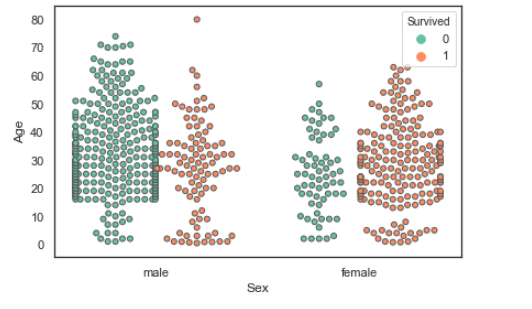## 箱线图

seaborn.boxplot(x=None, y=None, hue=None, data=None, order=None, hue_order=None, orient=None, color=None, palette=None, saturation=0.75, width=0.8, dodge=True, fliersize=5, linewidth=None, whis=1.5, notch=False, ax=None, **kwargs)

x, y, hue：数据或向量数据中的变量名称，可选用于绘制长格式数据的输入。查看样例以进一步理解。

data：DataFrame，数组，数组列表，可选用于绘图的数据集。如果x和y都缺失，那么数据将被视为宽格式。否则数据被视为长格式。

order, hue_order：字符串列表，可选控制分类变量（对应的条形图）的绘制顺序，若缺失则从数据中推断分类变量的顺序。

orient：“v” | “h”，可选控制绘图的方向（垂直或水平）。这通常是从输入变量的dtype推断出来的，但是当“分类”变量为数值型或绘制宽格式数据时可用于指定绘图的方向。

color：matplotlib颜色，可选所有元素的颜色，或渐变调色板的种子颜色。

palette：调色板名称，列表或字典，可选用于hue变量的不同级别的颜色。可以从 color_palette() 得到一些解释，或者将色调级别映射到matplotlib颜色的字典。

saturation：float，可选控制用于绘制颜色的原始饱和度的比例。通常大幅填充在轻微不饱和的颜色下看起来更好，如果您希望绘图颜色与输入颜色规格完美匹配可将其设置为1。

width：float，可选不使用色调嵌套时完整元素的宽度，或主要分组变量一个级别的所有元素的宽度。

dodge：bool，可选使用色调嵌套时，元素是否应沿分类轴移动。

fliersize：float，可选用于表示异常值观察的标记的大小。

linewidth：float，可选构图元素的灰线宽度。

whis：float，可选控制在超过高低四分位数时IQR的比例，因此需要延长绘制的触须线段。超出此范围的点将被识别为异常值。

notch：boolean，可选是否使矩形框“凹陷”以指示中位数的置信区间。还有其他几个参数可以控制凹槽的绘制方式；参见 plt.boxplot 以查看关于此问题的更多帮助信息。

ax：matplotlib轴，可选绘图时使用的Axes轴对象，否则使用当前Axes轴对象。

kwargs：键，值映射其他在绘图时传给 plt.boxplot 的参数。

sns.boxplot(x=data['Age'], whis=np.inf)
sns.stripplot(data['Age'], color='r')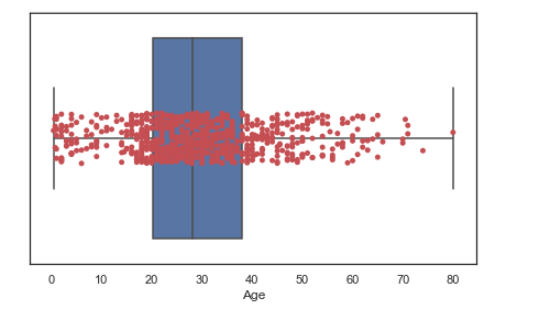sns.boxplot(x=data['Age'], y=data['Sex'], hue=data['Survived'], palette="Set3", linewidth=2.5,
order=["female", "male"], orient="h")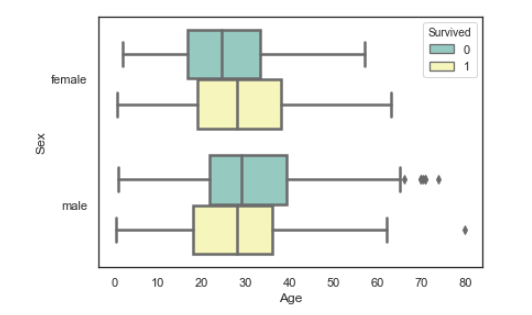sns.boxplot(x=data['Age'], y=data['Sex'], hue=data['Survived'], palette="Set3", linewidth=2.5,
order=["female", "male"], orient="h", dodge=False)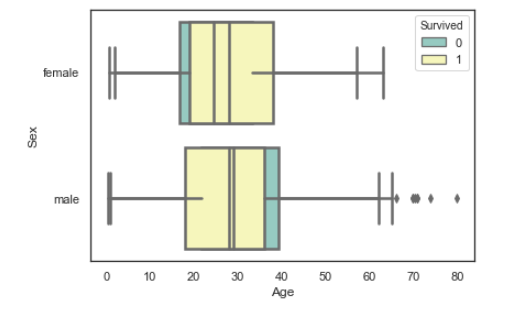### catplot()

sns.catplot(x="Sex", y="Age", hue="Survived", col="SibSp", data=data, kind="box", height=4, aspect=.7)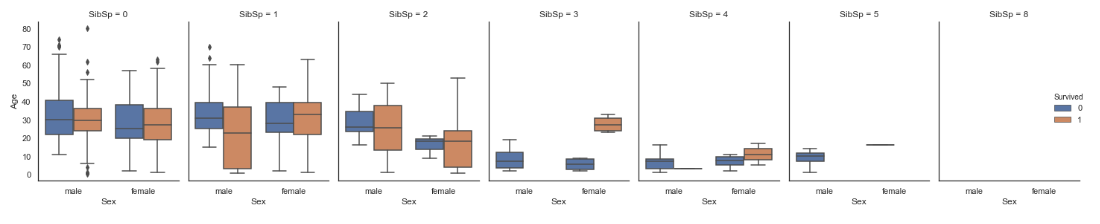## 散点图矩阵

seaborn.pairplot(data, hue=None, hue_order=None, palette=None, vars=None, x_vars=None, y_vars=None, kind=‘scatter’, diag_kind=‘auto’, markers=None, height=2.5, aspect=1, dropna=True, plot_kws=None, diag_kws=None, grid_kws=None, size=None)

pairplot方法实现数据特征的两两对比。默认是所有特征，可以通过vars参数指定部分特征。

# hue='列名'，通过绘图元素的颜色显示不同级别的分类变量
#sns.pairplot(data, hue='Survived', markers=["s", "D"])

sns.pairplot(data, vars=['Sex', 'Age', 'Survived'], height=3)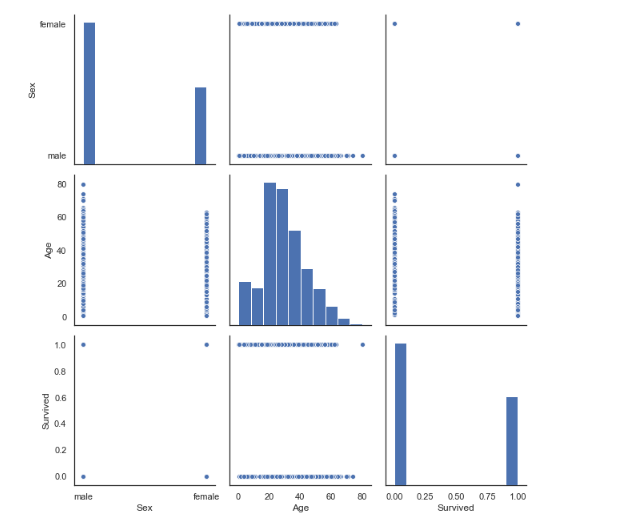sns.pairplot(data, x_vars=["Sex", "Age"], y_vars=["SibSp", "Parch"], hue='Survived', height=4)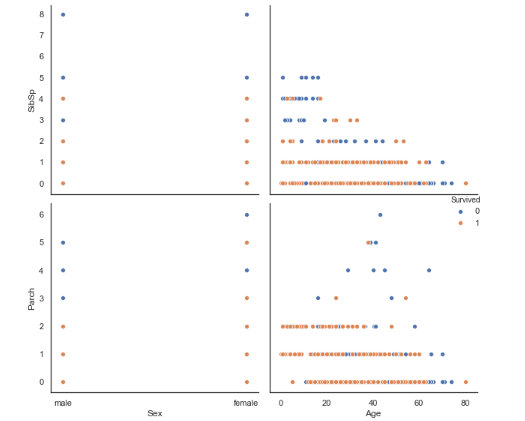#对单变量图使用核密度估计
#sns.pairplot(data, diag_kind="kde")

# 将线性回归模型拟合到散点图
#sns.pairplot(data, kind="reg")

# 将关键字参数传递给底层函数（直接使用PairGrid可能更容易）
sns.pairplot(data, markers="+", plot_kws=dict(s=50, edgecolor="b", linewidth=1))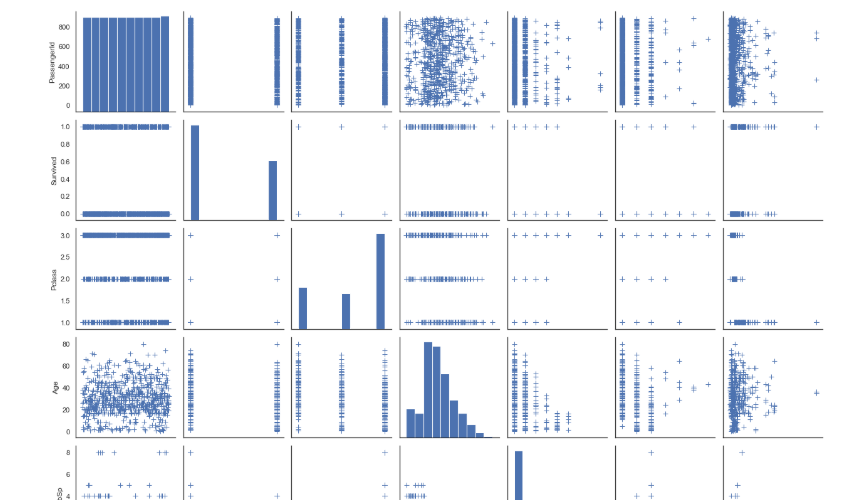## 小提琴图

seaborn.violinplot(x=None, y=None, hue=None, data=None, order=None, hue_order=None, bw=‘scott’, cut=2, scale=‘area’, scale_hue=True, gridsize=100, width=0.8, inner=‘box’, split=False, dodge=True, orient=None, linewidth=None, color=None, palette=None, saturation=0.75, ax=None, **kwargs)

x, y, hue：数据或向量数据中的变量名称，可选用于绘制长格式数据的输入。查看样例以进一步理解。

data：DataFrame，数组，数组列表，可选用于绘图的数据集。如果x和y都缺失，那么数据将被视为宽格式。否则数据被视为长格式。

order, hue_order：字符串列表，可选控制分类变量（对应的条形图）的绘制顺序，若缺失则从数据中推断分类变量的顺序。

bw：{‘scott’, ‘silverman’, float}，可选内置变量值或浮点数的比例因子都用来计算核密度的带宽。实际的核大小由比例因子乘以每个分箱内数据的标准差确定。

cut：float，可选以带宽大小为单位的距离，以控制小提琴图外壳延伸超过内部极端数据点的密度。设置为0以将小提琴图范围限制在观察数据的范围内。（例如，在 ggplot 中具有与 trim=True 相同的效果）

scale：{“area”, “count”, “width”}，可选该方法用于缩放每张小提琴图的宽度。若为 area ，每张小提琴图具有相同的面积。若为 count ，小提琴的宽度会根据分箱中观察点的数量进行缩放。若为 width ，每张小提琴图具有相同的宽度。

scale_hue：bool，可选当使用色调参数 hue 变量绘制嵌套小提琴图时，该参数决定缩放比例是在主要分组变量（scale_hue=True）的每个级别内还是在图上的所有小提琴图（scale_hue=False）内计算出来的。

gridsize：int，可选用于计算核密度估计的离散网格中的数据点数目。

width：float，可选不使用色调嵌套时的完整元素的宽度，或主要分组变量的一个级别的所有元素的宽度。

inner：{“box”, “quartile”, “point”, “stick”, None}，可选控制小提琴图内部数据点的表示。若为box，则绘制一个微型箱型图。若为quartiles，则显示四分位数线。若为point或stick，则显示具体数据点或数据线。使用None则绘制不加修饰的小提琴图。

split：bool，可选当使用带有两种颜色的变量时，将split设置为True则会为每种颜色绘制对应半边小提琴。从而可以更容易直接的比较分布。

dodge：bool，可选使用色调嵌套时，元素是否应沿分类轴移动。

orient：“v” | “h”，可选控制绘图的方向（垂直或水平）。这通常是从输入变量的dtype推断出来的，但是当“分类”变量为数值型或绘制宽格式数据时可用于指定绘图的方向。

linewidth：float，可选构图元素的灰线宽度。

color：matplotlib颜色，可选所有元素的颜色，或渐变调色板的种子颜色。

palette：调色板名称，列表或字典，可选用于hue变量的不同级别的颜色。可以从 color_palette() 得到一些解释，或者将色调级别映射到matplotlib颜色的字典。

saturation：float，可选控制用于绘制颜色的原始饱和度的比例。通常大幅填充在轻微不饱和的颜色下看起来更好，如果您希望绘图颜色与输入颜色规格完美匹配可将其设置为1。

ax：matplotlib轴，可选绘图时使用的Axes轴对象，否则使用当前Axes轴对象。

# palette="Set2"   inner="stick"
sns.violinplot(x='Sex', y='Age', hue='Survived', data=data, palette="muted", split=True,
order=["female", "male"], scale="count", inner="quartile", scale_hue=False, bw=.2, height=4)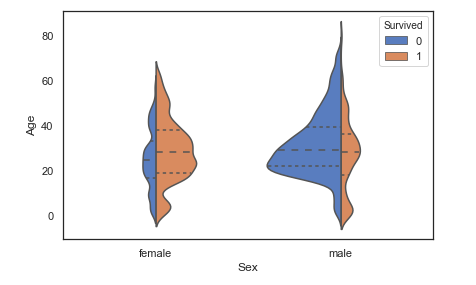## 条形图

seaborn.barplot(x=None, y=None, hue=None, data=None, order=None, hue_order=None, estimator=, ci=95, n_boot=1000, units=None, orient=None, color=None, palette=None, saturation=0.75, errcolor=’.26’, errwidth=None, capsize=None, dodge=True, ax=None, **kwargs)

data：DataFrame，数组或数组列表，可选绘图数据集。如果x和y都不存在，则解释为宽格式。否则，它将是长格式。

order, hue_order：字符串列表，可选为了绘制分类级别，否则从数据对象推断级别。

estimator：可调用映射向量->标量，可选统计函数，用于估计每个分类箱内。

ci：float或“ sd”或无，可选置信区间的大小，可用于估计值附近。如果为“ sd”，则跳过引导程序并绘制观测值的标准偏差。如果为None，则不会执行自举，并且不会绘制错误栏。

n_boot：int，可选计算置信区间时要使用的引导程序迭代次数。

units：变量data或向量数据的名称，可选采样单位的标识符，将用于执行多级引导程序并考虑重复测量的设计。

orient：“ v” | “ h”，可选绘图的方向（垂直或水平）。这通常是根据输入变量的dtype推断出来的，但可用于指定“分类”变量是数字时还是绘制宽格式数据时。

color：matplotlib颜色，可选所有元素的颜色，或渐变调色板的种子。

palette：调色板名称，列表或字典，可选用于hue变量的不同级别的颜色。应该是可以解释的内容color_palette()，或者是将色调级别映射到matplotlib颜色的字典。

saturation：浮动，可选原始饱和度的绘制颜色比例。大型色块通常看起来略带不饱和的颜色会更好，但是1如果您希望绘图颜色与输入颜色规格完全匹配，请将其设置为。

errcolor：matplotlib颜色代表置信区间的线条颜色。

errwidth：浮动，可选误差线（和顶盖）的粗细。

capsize：浮动，可选误差条上“盖子”的宽度。

dodge：布尔型，可选使用色相嵌套时，是否应沿分类轴移动元素。

ax：matplotlib轴，可选轴对象以绘制绘图，否则使用当前轴。

kwargs：键，值映射其他关键字参数plt.bar在绘制时传递给。

from numpy import median
# ci=68  palette="Blues_d"  dodge=False
sns.barplot('Sex', 'Age', hue='Survived', data=data, order=['female', 'male'], estimator=median, ci='sd',
capsize=.2, saturation=.5, facecolor=(1, 1, 1, 0), errcolor=".2", edgecolor=".2")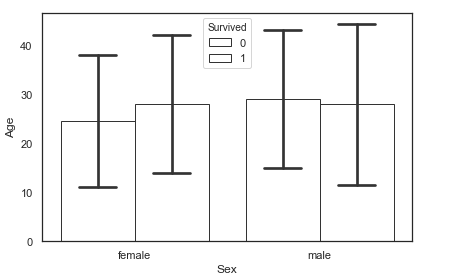### catplot()

sns.catplot(x="Sex", y="Age", hue="Survived", col="Pclass", data=data, kind="bar", height=4, aspect=.7)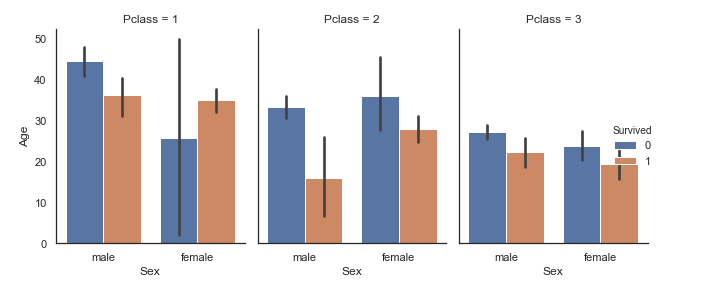### 使用条形显示每个分箱器中的观察计数countplot

seaborn.countplot(x=None, y=None, hue=None, data=None, order=None, hue_order=None, orient=None, color=None, palette=None, saturation=0.75, dodge=True, ax=None, **kwargs)

data：DataFrame，数组或数组列表，可选绘图数据集。如果x和y都不存在，则解释为宽格式。否则，它将是长格式。

order, hue_order：字符串列表，可选为了绘制分类级别，否则从数据对象推断级别。

orient：“ v” | “ h”，可选绘图的方向（垂直或水平）。这通常是根据输入变量的dtype推断出来的，但可用于指定“分类”变量是数字时还是绘制宽格式数据时。

color：matplotlib颜色，可选所有元素的颜色，或渐变调色板的种子。

palette：调色板名称，列表或字典，可选用于hue变量的不同级别的颜色。应该是可以解释的内容color_palette()，或者是将色调级别映射到matplotlib颜色的字典。

saturation：浮动，可选原始饱和度的绘制颜色比例。大型色块通常看起来略带不饱和的颜色会更好，但是1如果您希望绘图颜色与输入颜色规格完全匹配，请将其设置为。

dodge：布尔型，可选使用色相嵌套时，是否应沿分类轴移动元素。

ax：matplotlib轴，可选轴对象以绘制绘图，否则使用当前轴。

kwargs：键，值映射其他关键字参数则传递给plt.bar。

sns.countplot(x='Sex', hue='Survived', data=data)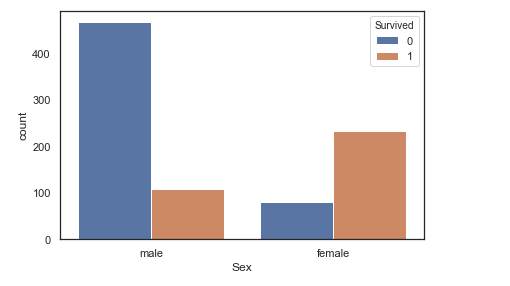sns.countplot(y="Pclass", hue="Sex", data=data, facecolor=(0, 0, 0, 0), linewidth=5,
edgecolor=sns.color_palette("dark", 3))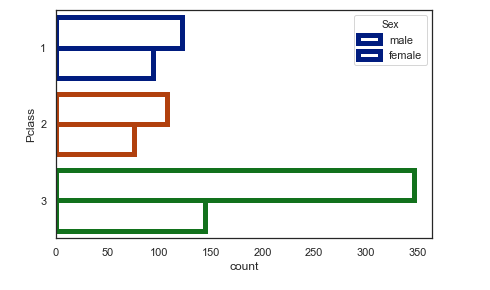## 多变量图

seaborn.jointplot(x, y, data=None, kind=‘scatter’, stat_func=None, color=None, height=6, ratio=5, space=0.2, dropna=True, xlim=None, ylim=None, joint_kws=None, marginal_kws=None, annot_kws=None, **kwargs)

### 用双变量和单变量图绘制两个变量的图。

x, y：strings or vectors中的数据或变量名称data。

data：DataFrame，可选DataFrame when x和y是变量名。

kind：{“分散” | “ reg” | “残渣” | “ kde” | “ hex”}，可选要绘制的情节。

stat_func：可调用或无，可选不推荐使用

color：matplotlib颜色，可选用于图元素的颜色。

height：数字，可选图的大小（将为正方形）。

ratio：数字，可选关节轴高度与边缘轴高度之比。

space：数字，可选关节轴和边缘轴之间的空间

dropna：布尔型，可选如果为True，请删除x和中缺少的观测值y。

{x, y}lim：二元组，可选绘制前要设置的轴限制。

{joint, marginal, annot}_kws：命令，可选绘图组件的其他关键字参数。

kwargs：键，值配对附加的关键字参数将传递到用于在关节轴上绘制图的函数，从而取代joint_kws字典中的项。

sns.set(style="white", color_codes=True)
# 添加回归和核密度拟合
sns.jointplot(x="Pclass", y="Age", kind='reg', data=data)  # 六边形箱将散点图替换为联合直方图kind="hex"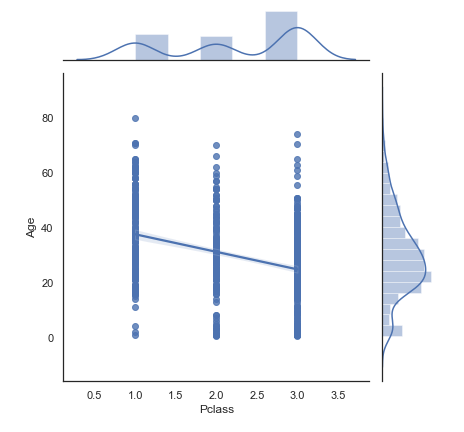# 用密度估计值替换散点图和直方图，并将边缘轴与关节轴紧密对齐：
sns.jointplot("Pclass", "Age", data=data, kind="kde", space=0, color="g")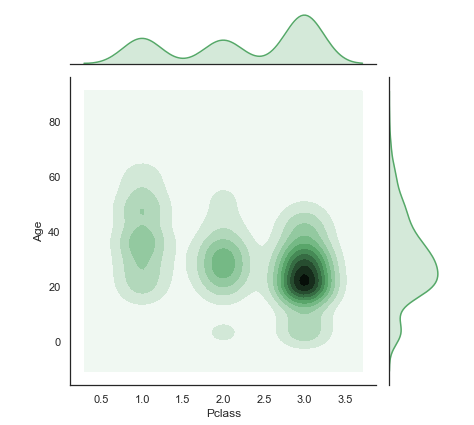# 绘制散点图，然后添加联合密度估计
sns.jointplot("Pclass", "Age", data=data, color="k").plot_joint(sns.kdeplot, zorder=0, n_levels=6)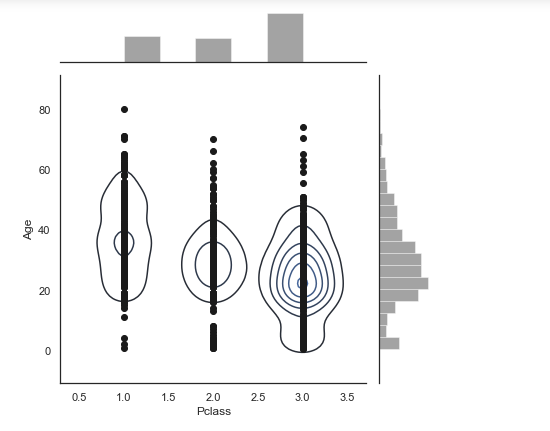sns.jointplot("Fare", "Age", data=data, marginal_kws=dict(bins=15, rug=True),
annot_kws=dict(stat="r"), s=40, edgecolor="w", linewidth=1)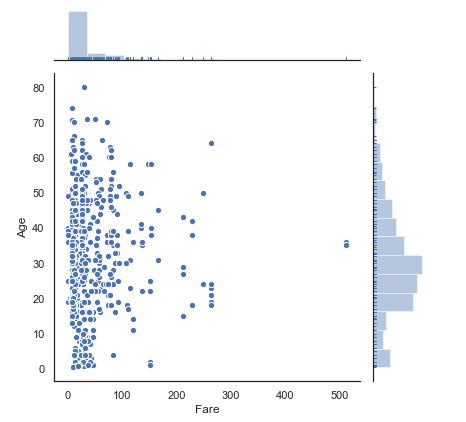## 回归图

seaborn.regplot(x, y, data=None, x_estimator=None, x_bins=None, x_ci=‘ci’, scatter=True, fit_reg=True, ci=95, n_boot=1000, units=None, order=1, logistic=False, lowess=False, robust=False, logx=False, x_partial=None, y_partial=None, truncate=False, dropna=True, x_jitter=None, y_jitter=None, label=None, color=None, marker=‘o’, scatter_kws=None, line_kws=None, ax=None)

x，y：字符串，序列或向量数组输入变量。如果是字符串，则这些字符串应与中的列名称相对应data。使用熊猫对象时，轴将用系列名称标记。

data：数据框整齐（“长格式”）数据帧，其中每一列都是变量，每一行都是观察值。

x_estimator：可调用映射向量->标量，可选将此函数应用于的每个唯一值，x并绘制得出的估计值。当x是离散变量时，这很有用。如果x_ci给出，该估计将被引导，并得出一个置信区间。

x_bins：int或矢量，可选将x变量分为离散的bin，然后估计中心趋势和置信区间。这种装仓仅影响散点图的绘制方式；回归仍然适合原始数据。此参数被解释为均匀大小（不必要间隔）的垃圾箱数或垃圾箱中心的位置。使用此参数时，表示默认x_estimator为numpy.mean。

x_ci：“ ci”，“ sd”，在[0，100]中为int或无，可选绘制离散值的集中趋势时使用的置信区间的大小x。如果为"ci"，则遵循ci参数的值。如果为"sd"，则跳过引导程序，并显示每个仓中观测值的标准偏差。

scatter：布尔型，可选如果为True，则绘制一个散点图以及基础观测值（或x_estimator值）。

fit_reg：布尔型，可选如果为True，则估计并绘制与x和y变量相关的回归模型。

ci：int in [0，100]或无，可选回归估计的置信区间的大小。这将使用回归线周围的半透明带绘制。置信区间是使用自举估算的；对于大型数据集，建议将此参数设置为“无”以避免计算。

n_boot：int，可选用于估计的自举重采样次数ci。默认值试图平衡时间和稳定性。您可能需要增加“最终”版图的此值。

units：变量名data，可选如果x和y观测值嵌套在采样单位内，则可以在此处指定。在通过执行对单元和观测值（单元内）都重新采样的多级引导程序来计算置信区间时，将考虑到这一点。否则，这不会影响回归的估计或绘制方式。

order：int，可选如果order大于1，则用于numpy.polyfit估计多项式回归。

logistic：布尔型，可选如果为True，则假定y为二进制变量，并用于statsmodels估计逻辑回归模型。请注意，这比线性回归的计算量大得多，因此您可能希望减少引导程序重采样（n_boot）的数量或将其设置ci为“无”。

lowess：布尔型，可选如果为True，则用于statsmodels估计非参数的最低模型（局部加权线性回归）。请注意，目前无法为这种模型绘制置信区间。

robust：布尔型，可选如果为True，则用于statsmodels估计稳健的回归。这将消除异常值的权重。请注意，这比标准线性回归的计算量大得多，因此您可能希望减少引导程序重采样（n_boot）的数量或将其设置ci为“无”。

logx：布尔型，可选如果为True，则估计形式为y〜log（x）的线性回归，但在输入空间中绘制散点图和回归模型。请注意，这x必须是积极的。

{x,y}_partial：字符串data或矩阵混淆变量以在绘制前从x或y变量中回归。

truncate：布尔型，可选默认情况下，绘制散点图后将绘制回归线以填充x轴限制。如果truncate为True，它将受到数据限制的限制。

{x,y}_jitter：浮标，可选将相同大小的均匀随机噪声添加到x或y变量中。拟合回归后，噪声会添加到数据副本中，并且只会影响散点图的外观。在绘制采用离散值的变量时，这将很有帮助。

label：串用于在图例中使用的散点图或回归线（如果scatter是False）应用于以太的标签。

color：matplotlib颜色颜色适用于所有绘图元素；将由scatter_kws或传入的颜色取代line_kws。

marker：matplotlib标记代码用于散点图字形的标记。

{scatter,line}_kws：词典传递给plt.scatter和的其他关键字参数plt.plot。

ax：matplotlib轴，可选轴对象以绘制绘图，否则使用当前轴。

sns.regplot(x="Fare", y="Age", data=data, marker="+", ci=68, x_jitter=.1)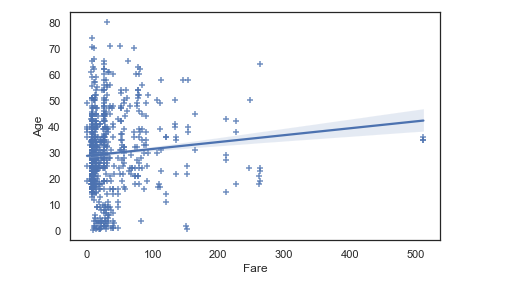sns.regplot(x="Fare", y="Age", data=data, x_bins=4)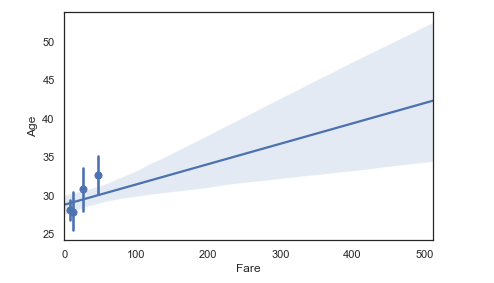09-1637307-17440
09-081201
05-301万+
04-24232
07-306829
12-01525
08-221192
08-302703
04-116692
06-1857
07-07314
01-061543
09-22341
02-27114
04-2749
07-10275
02-042900点击重新获取扫码支付余额充值